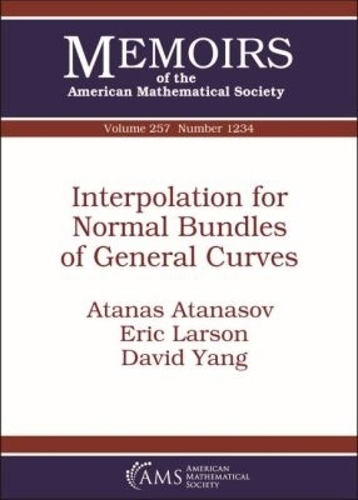•Interpolation for Normal Bundles of General Curves - Memoirs of the American Mathematical Society (Paperback)

(author), (author), (author)
£82.50
Paperback 105 Pages / Published: 30/12/2018
• We can order this

Usually dispatched within 2 weeks

Given $n$ general points $p_1, p_2, \ldots , p_n \in \mathbb P^r$, it is natural to ask when there exists a curve $C \subset \mathbb P^r$, of degree $d$ and genus $g$, passing through $p_1, p_2, \ldots , p_n$. In this paper, the authors give a complete answer to this question for curves $C$ with nonspecial hyperplane section. This result is a consequence of our main theorem, which states that the normal bundle $N_C$ of a general nonspecial curve of degree $d$ and genus $g$ in $\mathbb P^r$ (with $d \geq g + r$) has the property of interpolation (i.e. that for a general effective divisor $D$ of any degree on $C$, either $H^0(N_C(-D)) = 0$ or $H^1(N_C(-D)) = 0$), with exactly three exceptions.

Publisher: American Mathematical Society
ISBN: 9781470434892
Number of pages: 105
Dimensions: 254 x 178 mm